# Noise Level Measurements in Amorphous & CRGO Core Distribution Transformers

The article explores the sources of noise in Amorphous and CRGO core distribution transformers and the ways in which it may be controlled.

Noise is generally defined as an unpleasant sound. The noise of range more than 70 dB is unacceptable to people residing near distribution transformers. The power utilities are continuously stipulating lower transformer noise pressure levels. Transformer noise comprises two components that is no-load noise and load noise. The sources of transformer noise can be divided into two categories:
• Sources of noise attributed to magnetostrictive forces acting on the core parts.
• Sources of noise attributed to magneto- motive forces acting on the core and structural parts.

The noise pressure generated by vibration of core and windings is transmitted to tank surfaces though the oil medium. Since the oil is relatively incompressible, the noise is transmitted without appreciable damping. The tank responds to these noise waves depending on its natural frequencies and made shapes of vibrations. When measuring sound pressure levels generated by a transformer, its emitted sound pressures are compared to a standardised noise pressure level as described in IEC 60076-10. The noise level is usually specified as an ‘A-weighted’ average of sound pressure levels measured at several frequencies.

Noise due to magnetostriction
Understanding core steel
Modern transformer cores are built from several sheets of stacked or wound energy-efficient grain- oriented electrical steel (GOES).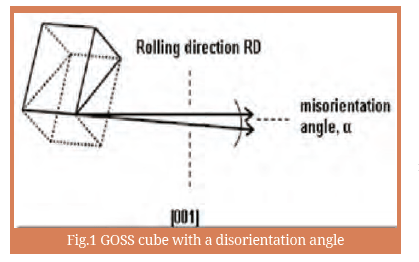This material is a ferromagnetic alloy of iron (Fe) with 3 per cent silicon (Si) content, and is characterised by low iron losses and magnetostriction. These properties are achieved through a series of intricate processes of hot and cold rolling of the sheets in such a way that the magnetic properties are optimised in the rolling direction. The end product has a sharp Grain Oriented Silicon Steel (GOSS) texture i.e. material grains are aligned along the edges of the GOSS cube, with a disorientation angle of approximately 3°, as shown in figure. 1.

Core magnetisation
The primary source of acoustic noise generation in a transformer is the periodic mechanical deformation of the transformer core and the winding coils. The periodic change in length of the core steel is termed magnetostriction and is a measure of the change in length over the original length of the material. The magnetostrictive force sets the core in vibration. These vibrations are transmitted through the fluid within the transformer oil or air, to the structural parts and through the tank wall into the surrounding air where they are heard as an audible noise. Factors that affect magnetostriction include the instantaneous value of the flux density and the material properties, as shown in Eqn.1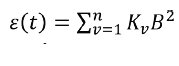…………………………………(1)
where:
ɛ(t) = the unit coefficient of magnetostriction
B = instantaneous flux density in Tesla
Kv = coefficient which depends on level of
magnetization, type of lamination material and treatment
thereof
The magnetostrictive force is given by Eqn.2: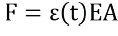……………………………………………….(2)
where:
F = magnetostrictive force in Newtons
ɛ(t) = the unit coefficient of magnetostriction
E = modulus of elasticity in Pascals
A = cross-sectional area of a lamination sheet in m2

The magnetostrictive force is a time-varying parameter that contains even harmonics of the fundamental frequency i.e. 100 Hz, 200 Hz, 300 Hz, for a 50 Hz supply, whereas the magnetostrictive noise varies with the magnetostrictive force which in turn varies with odd harmonics of the fundamental frequency i.e. 100 Hz, 300 Hz, 500 Hz, 700 Hz . Numerous studies show that transformers designed to operate at flux densities well below saturation have lower noise emissions than those operating near saturation. This is because at saturation, all the domains have reoriented themselves in the applied field and there is no further rotation. The same behavior can be seen in the grades of amorphous core material also. The saturation flux density in the HBI grade of amorphous core is around 1.65 Tesla where as in the SA1 grade of amorphous core is of around 1.58 Tesla. The application of amorphous grade SA1M is superior to SA1 in terms of Nosie level reduction and other magnetic properties. The B-H curves between amorphous grade materials are shown in the figure.2 below. The amorphous core is very much significant in reducing the core losses to maximum extent but not in the noise level reductions at certain cases.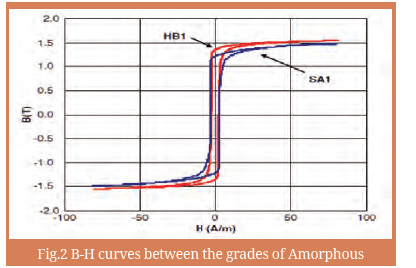• The material of a transformer core exhibits   magnetostrictive properties. The vibration of the core is due to its magnetostrictive strain varying at twice the frequency of the alternating magnetic flux. The frequencies of the magnetic flux are equal to the power system supply frequency and its harmonics.
• When there are residual gaps between laminations of the core, the periodic magneto-motive force may cause the core laminations to strike against each other and produce noise. Also, the periodic mutual forces between the current-carrying coil windings can induce vibrations if there are any loose turns of the coil.

The no-load noise measured in ‘dB’ for CRGO core transformers are not of much variation from position to position on prescribing contour irrespective of rating of the transformer. In the Anechoic chamber, the noise values measured are almost uniform in kind around the prescribing contour drawn. But it is not the case for amorphous core transformers. We can observe the considerable changes in values of noise measured at the various positions on prescribing contour. The finishing of the core assembly with reference to workmanship is very crucial for the amorphous core designs. The overall A-weighted noise measured in the amorphous core transformers are significantly more than the CRGO core transformers of same rating. The results have been highlighted in the subsequent portions of this paper. The noise is emitted by a loaded transformer in addition to its no-load noise.

It is caused by electromagnetic forces between the windings resulting from the leakage fields and is proportional to the square of the load current. These forces cause the winding vibrations and acoustic radiations having frequency of 100 or 120 Hz that is twice the power frequency. The contribution of load-controlled noise to the overall noise level of the transformer becomes significant even if the operating flux density in the core is lower. Generally, at lower value of flux density, noise from the core is considerably reduced.

Noise level measurements of Transformers
A noise level is commonly measured in decibels (dB) by comparing the pressure generated by a noise source with some standard level. The noise level is measured basically two methods, sound pressure measurement and sound intensity measurement. The details of test methods and acceptable test environment conditions are given in standard IEC: 60076–10. The methods are applicable to transformers, reactors and their cooling devices. Where conditions are close to freefield, essentially undisturbed by reflections from nearby objects and the environment boundaries, as sometimes achieved for outdoor measurements, then the value for ‘K’ would tend to zero and no environmental correction is necessary. IEC 60076- 10 requires all sound measurements to be made over a reflecting surface. Therefore, measurements in fully anechoic chambers are not necessary. The measurements in Semi-anechoic Chamber are shown in figure.3.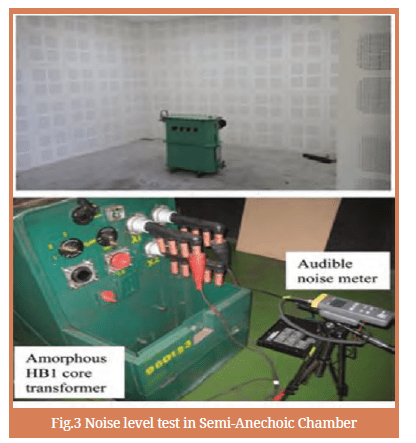Noise level reduction techniques

As the noise has become one of the key issues, it should be addressed starting from the design and other stages by the effective techniques. A lower value of the operating flux density also results in higher material cost and size of the transformer. Hence, other cost-effective noise reduction methods are commonly used which are now described. The noise reduction techniques can be summarised as below: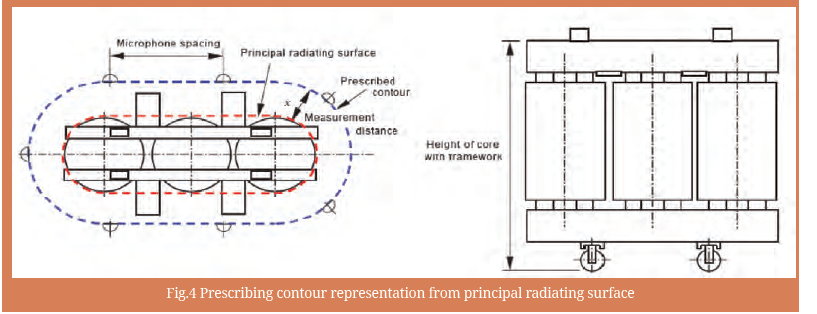• Reduction in core flux density: This gives noise reduction of 3 to 5 dB for a reduction in flux density by 10 per cent or approximately 2 dB per flux density reduction of 0.1 T. The method has adverse effects on the cost and size of DTRs.
• Hi-B grade and scribed core materials give 2 to 3 dB reduction as compared to non-Hi-B grades.
• Increased core damping: By application of suitable viscoelastic or adhesive coating to the core laminations, the noise level can be reduced. It should be ensured that any links or attachments to the core are flexible so that they do not transmit the vibrations.
• Use of step-lap joint: It gives reduction by about 4 to 5 dB as compared to the mitred construction for the commonly used flux densities (1.6 to 1.7 T). The corner protrusions of the built core should be cut since they may contribute to noise due to vibrations.
• To reduce the structure borne vibrations, the corewinding assembly should be isolated from the tank base by use of oil compatible anti-vibration pads between them. Use of anti-vibration pads is also made between frames and tank. Such isolations can give a noise level reduction of 2 to 4 dB.
• Use of sound insulation panels between tank stiffeners can give 5 to 15 dB reduction. An increased tank wall mass, by use of sand in hollow braces on the wall, can give appreciable noise level reduction.
• Use of double tank design: Inner and outer tanks are suitably insulated from each other to eliminate structure borne vibrations. Also, suitable sound absorbent wool is placed between the two tanks. The noise reduction is about 15 dB.

Test Conditions
Environment
Measurements should be made in an environment having an ambient sound pressure level at least five decibels below the combined sound pressure level of the transformer and the ambient sound pressure level. For one-third octave or narrow band measurements, the five-decibel difference shall apply to each frequency band in which measurements are being made. When ambient sound conditions do not comply with the above, suitable corrections may be feasible when the ambient sound conditions are steady and discrete frequency sound levels are measured. The environmental correction factor ‘K’ shall preferably be determined by measurement techniques. However, for the purpose of this standard estimation for ‘K’ is allowed by use of absorption coefficients. In order to account for the effect of these sound reflections, the IEC Standards provides the following calculation formula for what is termed as the “Environmental factor K”. The calculated value as per equations (3) & (4) is to be subtracted from the measured value of the noise level.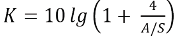………………………………..(3)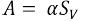………………………………..(4)
Where:
α = Average Acoustic Absorption coefficient (Table–1)
Sv= Total Area of the Surface of the test room
S = Transformer Measurement Surface Area
The maximum value allowed for this Environmental factor correction is 7 dB, otherwise measurements would be considered invalid. For a test room to be satisfactory, A/S shall be ≥1. This will give a value for the environmental correction factor K of ≤7 dB.

Calculation of average sound pressure level
The uncorrected average A-weighted sound pressure level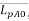, shall be calculated from the A-weighted sound
pressure levels LpAi, measured with the test objectenergized by using equation as per equation (5).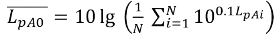………………..(5)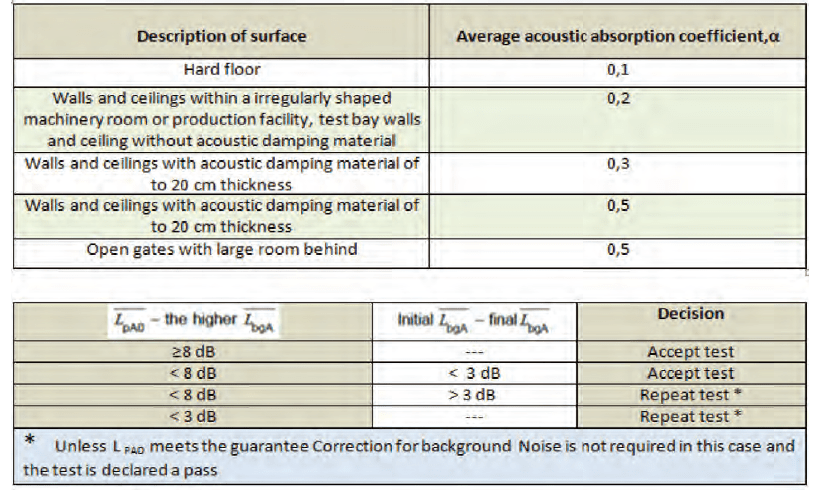Where N is the total number of measuring positions. The average A-weighted background noise pressure level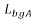, shall be calculated separately before and after the test sequence using equation (6).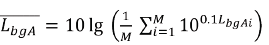………………..(6)

Where M is the total number of measuring positions LbgAi is the measured A-weighted background noise pressure level at the ith measuring position.

If the initial and final average background noise pressure levels differ by more than 3 dB and the higher value is less than 8 dB lower than the uncorrected average A-weighted sound pressure level, the measurements shall be declared invalid and the test repeated except in cases where the uncorrected average A-weighted sound pressure level is less than the guaranteed value. In this case, the test object shall be considered to have met the guaranteed level. While the standard permits a small difference between the background noise level and the combined sound level of the background and the test object, every effort should be made to obtain a difference of at least 6 db. The corrected average A-weighted sound pressure level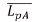, shall be calculated by using equation (7).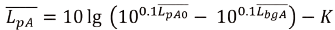…….(7)

where is the lower of the two calculated average A-weighted background noise pressure levels. As per the standard, the maximum allowable value of the environmental correction ‘K’ is 7 db. The A-weighted sound power level of the test object, LWA, shall be calculated from the corrected average A-weighted sound pressure level,according to equation (8).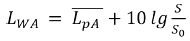…………………………………..(8)

where 𝑆 is derived from the following equation (9) and 𝑆0 is equal to the reference area (1 m2). The area 𝑆 of the measurement surface, expressed in square meters, is given by

Where
h is the height in meters of the transformer tank lm is the length in meters of the prescribed contour. 1.25 is an empirical factor intended to take account of the sound energy radiated by the upper part of the test object as shown in figure.4

Noise Level measurements and Analysis on Amorphous and CRGO cores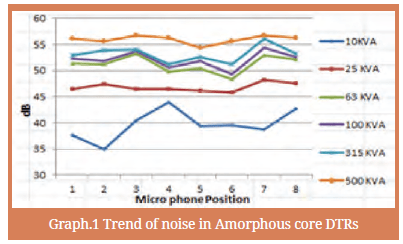The noise level results on amorphous core transformers of various ratings up to 500 kVA are shown in the Graph 1. The comparison between noise level between amorphous and CRGO transformers for various ratings is given in the Graph 2 shown above. The noise values are not of uniform in nature around the prescribing contour drawn. For the ratings of 63 kVA and 100 kVA the noise trend of Amorphous versus CRGO is shown in Graph 3 & Graph 4 from the two different manufacturers, The amorphous core is exhibiting more noise values.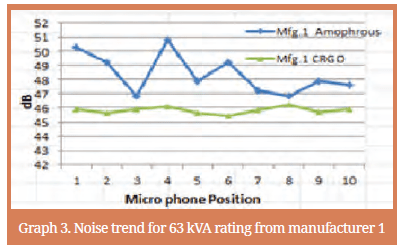Effect of short Circuit on Noise level Results The noise level results on various transformers have proven that that there is effect of Short- time Current (STC) on the transformers for exhibiting the higher noise levels. Presently amorphous core transformers of rating up to 1 MVA are in market. The noise level measurements before and after the short circuit test has shown considerable increase of noise up to 5 dB especially in amorphous core transformers. This has been illustrated in the Graph.6 for 100 kVA amorphous core distribution transformer. This effect is mainly due to the following listed out causes which are outcome of the Short circuit with stand test which is of distractive in nature on transformers. The figure 5 a) & b) illustrates this phenomenon.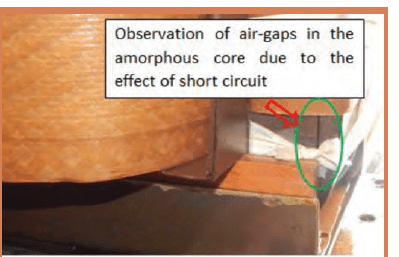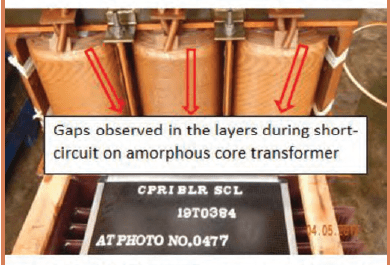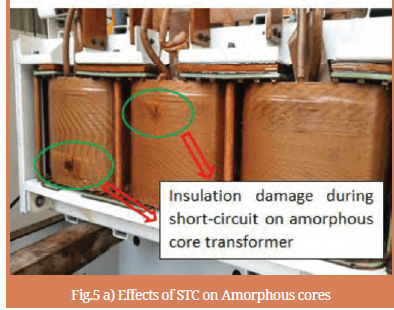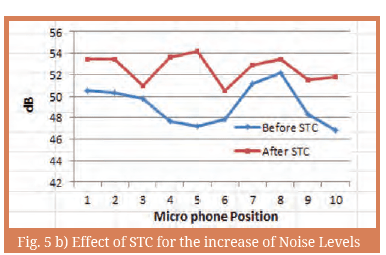• The short circuit test may result in creation smallair gaps in the core of transformers designed with amorphous core.
• Gaps may get form on the layers of the winding due the effect of short- circuit on amorphous core which results to higher noise.
• The isolation damage occurred during short circuit test may results in rise the values of noise levels measured.

Limits of sound power level
The distribution transformers noise level should not exceed the values specified in accordance with the conditions outlined in IEC, NEMA, ANSI/IEEE C57.12.90-1993 Standards and the limits for the oil cooled transformers up to 2500 kVA are listed out in the table 2.National Electrical Manufactures Association (NEMA) Standards publication No.TR1-1993 (R2000) is specified the limits for noise levels both for oil cooled and dry type transformers with nominal system voltage up to 15 kV.

Conclusions
In addition to the design challenges, the measurement of low noise poses a difficult problem. The minimum level of noise which can be measured is limited by the ambient noise conditions in the test area. Special enclosures may have to be used to shield the instruments and transformer from the high ambient noise. Sound reduction methods have been explored in various literatures. However, in order to make these methods to be effective, the transformer manufacturer needs to understand completely the nature and origin of transformer noise and should be able to handle through proper selection of the core and flux density. Amorphous core transformers are giving considerable high nose than CRGO. The effect of short circuit is significant in making the analysis of Noise level on Amorphous core transformers. Accurate measuring and calculating techniques as well as detailed modelling of the transformer are critical considerations when designing transformers with low noise emission.S. Arjuna Rao
Engineering
Officer., CPRISwaraj Kumar Das,
Director, CPRIG. Girija,
Joint Director,
CPRI, Bangalore.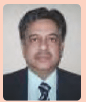R. A. Deshpande,
Director, CPRIB. R. Vasudevamuthy
Joint Director, CPRI

#### 1 COMMENT

1. Please let us have your kind offer for 1 pc Sound level meter for power transformer .

Thank you

Sincerely yours,